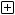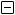GeneralRenaming methodsTagsUsing Advanced RenamerSettings dialogImport

Display in one page

# Renumber methodThis method can be used to change existing numbers in file names. You cannot use this method to add new numbers, for that use the New Name or Add method.

Number position
This field decides which number in the filename you want to change. If the filename is "File_004_A_202.txt" and you set it to 2 it is the number 202 that will get changed.

Change to
The change to drop down box is used to switch between the two renumbering modes.
Absolute number is used when you want to completely renumber the files at a given start number. The New Number field decides the number for the first file.
Relative number is used when you want to add or subtract a value from the existing number. This can be handy if you have a sequence of files with gaps between some of the numbers and you want to preserve this gap. This is commonly used when a sequence starts at 0 and you want to change it to start at 1 instead.

New number (only Absolute number mode)
Defines the number of the first file. Subsequent files will get their number calculated based on this number and the index of the file in the list.

Number difference (only Relative number mode)
If you want to add 3 to the number of all files in the list set this text field to 3. If you want to subtract three from the numbers type -3 instead.

Skip (only Absolute number mode)
If set to 1 the resulting names will be 001, 002, 003, 004. If set to 2 the resulting numbers will  be 001, 003, 005, 007.